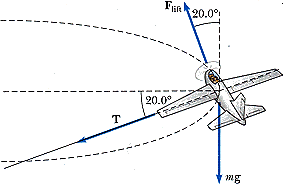# Model Airplane: Tension/Circular Motion/Lift (1 Viewer)

### Users Who Are Viewing This Thread (Users: 0, Guests: 1)

#### phy221

1. The problem statement, all variables and given/known data

A model airplane of mass 0.710 kg flies in a horizontal circle at the end of a 63.0 m control wire, with a speed of 35.0 m/s. Compute the tension in the wire if it makes a constant angle of 20.0° with the horizontal. The forces exerted on the airplane are the pull of the control wire, the gravitational force, and aerodynamic lift, which acts at 20.0° inward from the vertical as shown in the figure.2. Relevant equations

Fnet = ma

3. The attempt at a solution

m = .710kg
v(horizontal) = 35m/s
mg = 6.958N
r = 63cos20 = 59.20m
a(radial) = (35^2)/59.20 = 20.69m/s^2
F(radial) = .710(20.69) = 14.6899 = 14.69N
F(vertical) = mg + Ty - Flift(y-component) = 6.958 + Tsin20 - Flift*sin20
F(horizontal) = F(radial)+ Tx = 14.69 + Tcos20

At this point I don't know how to use the lift to calculate the vertical force, and otherwise cannot find the tension components.

My book does not include any examples of this kind of problem, and the only match I found referred me back here to an unanswered post.

#### Attachments

• 5.1 KB Views: 333

#### tiny-tim

Homework Helper
welcome to pf!

hi phy221! welcome to pf!(try using the X2 icon just above the Reply box)

You don't know Flift, so taking horizontal and vertical components of F = ma won't help much

try some other direction for F = ma### The Physics Forums Way

We Value Quality
• Topics based on mainstream science
• Proper English grammar and spelling
We Value Civility
• Positive and compassionate attitudes
• Patience while debating
We Value Productivity
• Disciplined to remain on-topic
• Recognition of own weaknesses
• Solo and co-op problem solving